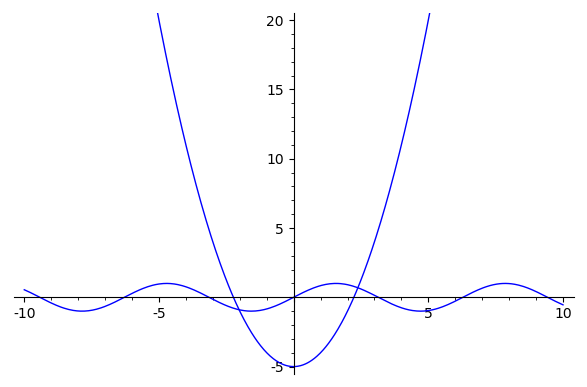Ask Your Question

# plot of sine, parabola intersection works but solve makes no sense

When I plot a sine and parabola I get two obvious intersections

plot(x^2-5,-10,10,ymax=20) + plot(sin(x),-10,10)


But when I solve to get the two numeric x values, the answer makes no sense.

solve(x^2-5==sin(x),x)
[x == -sqrt(sin(x) + 5), x == sqrt(sin(x) + 5)]edit retag close merge delete

## 1 Answer

Sort by » oldest newest most votedIt makes sense: it tried to solve symbolically, and it didn't get very far (it isolated the square, and took ± the square root).

You don't want to solve symbolically but numerically. You want to find the two real zeros of x^2 - 5 - sin(x), and from the picture you (roughly) know intervals where these are located, so you can use find_root:

sage: find_root(x^2 - 5 - sin(x), -5, 0)
-2.025211637444818
sage: find_root(x^2 - 5 - sin(x), 0, 5)
2.3846766601465696

more

## Comments

Thanks. Although it's odd that plot can "solve" it but solve cannot. Maybe in a future iteration of sagemath, a symbolic failure will default to find_root.

Can you only get this numerically or is there some form of advanced math that could solve a trig/algebra equivalence? I couldn't think of a way to do it by hand.

Most equations have no clean solution and must be evaluated with numerical approximations. This is one of them. Even polynomials can cause problems.

## Your Answer

Please start posting anonymously - your entry will be published after you log in or create a new account.

Add Answer

## Stats

Asked: 2020-10-18 08:14:31 +0200

Seen: 63 times

Last updated: Oct 18 '20